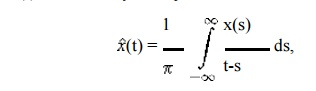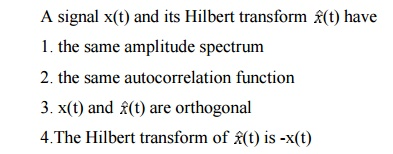Home | | Communication Theory | Hilbert Transform

# Hilbert Transform

A Hilbert transformer produces a -90 degree phase shift for the positive frequency components of the input x(t), the amplitude doesn't change.

HILBERT TRANSFORM:where the integral is the Cauchy principal value integral. The reconstruction formuladefines the Hilbert inverse transform.

ü  Hilbert transformer:A Hilbert transformer produces a -90 degree phase shift for the positive frequency components of the input x(t), the amplitude doesn't change.

ü   Properties of the Hilbert transform:ü   Pre envelope:

The pre envelope of a real signal x(t) is the complex functionThe pre envelope is useful in treating band pass signals and systems. This is due to the resultü   Complex envelope:

The complex envelope of a band pass signal x(t) isStudy Material, Lecturing Notes, Assignment, Reference, Wiki description explanation, brief detail
Communication Theory : Amplitude Modulation : Hilbert Transform |